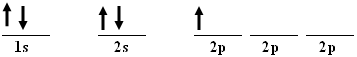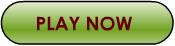# It's Elementary :

## Notation Styles

 Chapter Pages ------------- 1 - Introduction 2 - Energy Levels 3 - Orbitals 4 - Notation Styles Activity: Building ElementsTool: Interactive Periodic TableStyle One Chemists commonly use two systems of notation to show the electron placement in an atom. The electron configuration of boron is 1s2 2s2 2p1 The 1 on the left tells you that the first energy level is being used. The type of orbital in the first energy level is an s orbital. It contains two electrons. The next energy level being filled is the second energy level, shown by the 2 in the notation. The first orbital to receive any electrons in this level is an s orbital. The 2s orbital contains two electrons (its maximum). The last electron to be placed in an atom of boron is also in the second energy level. This electron is placed in the first p orbital. All the elements can have their electron arrangements listed in this way. The total of the superscripts will always be equal to the atomic number of the element when the notation is properly written. Style Two The second system of notation is called orbital notation. The electrons are drawn in on blanks representing the orbitals. Each electron is represented as either an up-facing arrow or a down-facing arrow. The order of the orbitals being filled can be determined by looking at the orbital chart below. The orbital notation for boron is shown.Note that the first arrow of each orbital is up-facing. Remember, according to Hund's Rule, each of the 2p orbitals will receive one electron (up-facing arrow) before any will get a second electron (down-facing arrow). The total number of arrows in the notation will equal the atomic number. You've now learned what you need to know in order to "build up" an atom from scratch. Let's test your new knowledge!